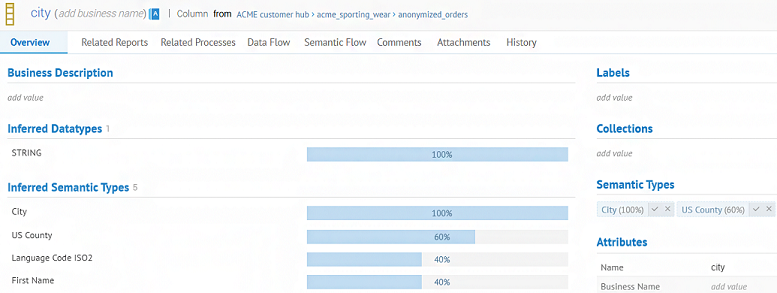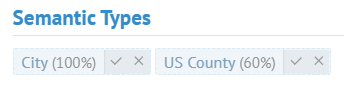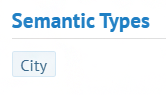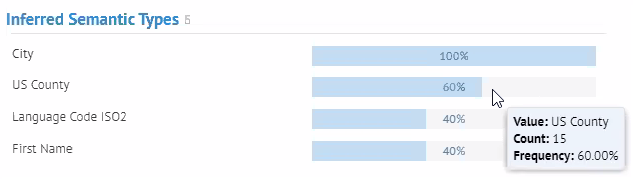# Discovering semantic and data types - Cloud

## Talend Cloud Data Catalog User Guide

EnrichVersion
Cloud
EnrichProdName
Talend Cloud
EnrichPlatform
Talend Data Catalog
Data Governance

The data discovery calculates how many values match each semantic type and, if the result is greater than 40%, it suggests the semantic type. The data discovery also assigns data types.

From the Overview tab, you can see the percentages in the Inferred Datatypes and Inferred Semantic Types areas.The inferred semantic types greater than 50% are suggested in the Semantic Types area.To assign or reject a proposed semantic type, click the tick or cross button. You can assign more than one semantic type. When a semantic type is rejected, it is removed.## How is the percentage calculated?

This percentage is the sum of two percentages:
• One percentage represents the number of values matching the semantic type; up to 100% allocated.

To determine if a value matches a semantic type, the data discovery depends on the type of the semantic type:

• Enumeration: Does the value match a value from the dictionary? Punctuation, case, spaces and accents are ignored.
• Regular expression: Does the value match the regular expression?
• Compound: is the value discovered into at least one child?
A compound type is a group of existing semantic types, called children.

If the answer is positive, the value is considered valid.

• The other percentage represents the similarity between the column name and the name of the semantic type; up to 10% allocated.
To compare the names:
• The Levenshtein algorithm is used. It calculates the minimum number of edits (insertion, deletion or substitution) required to transform one string into another.
• The case and accents are ignored.
• If the strings contain spaces, the word order is ignored. For example, US Phone and Phone US are considered identical.
The maximum percentage is 100%. If all values match a semantic type and the column name is identical to the name of the semantic type, the result still is 100%.

## More details from the quality bar

The quality bar shows the percentage of valid values according to the semantic types. To see the exact number of valid values, hover over the quality bar.## Discovering data types

Data types are automatically assigned. You do not need to accept them.

To determine of which type is a value, the data discovery follows an order:
1. Is the value empty?
2. Is the value of type boolean? true and false are the only values considered of type boolean.
3. Is the value of type integer?
4. Is the value of type decimal?
5. Is the value of type date?
6. If the value is not of one of the above types, it is considered a text value.

As the verification is incremental, a value is only of one type. For example, the value 5 is of type integer. It will not be considered of type text.